Successfully reported this slideshow.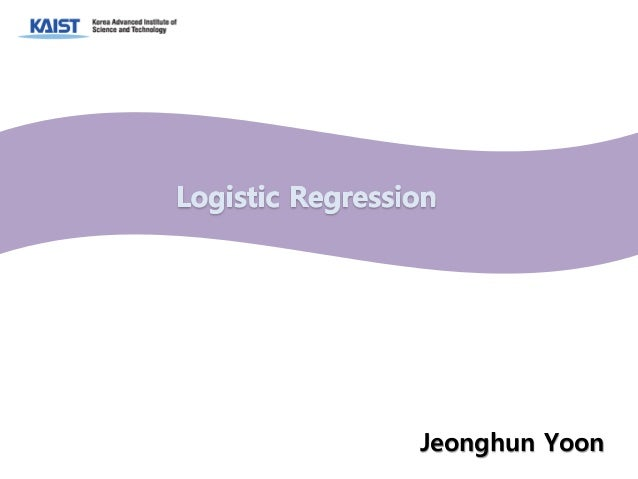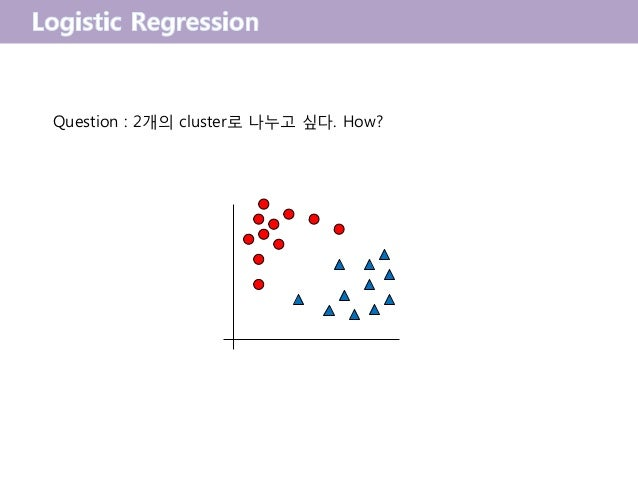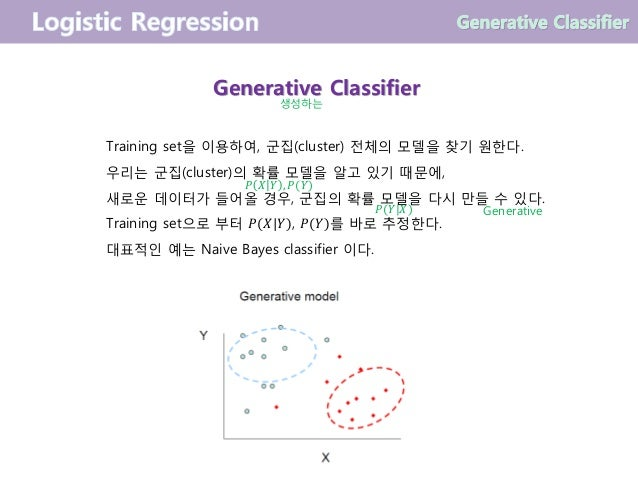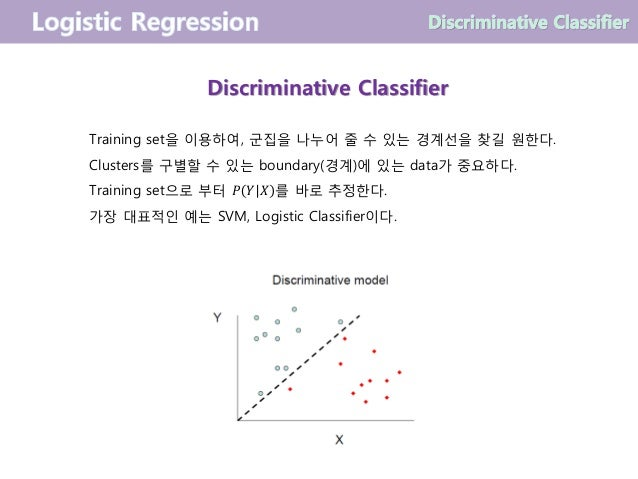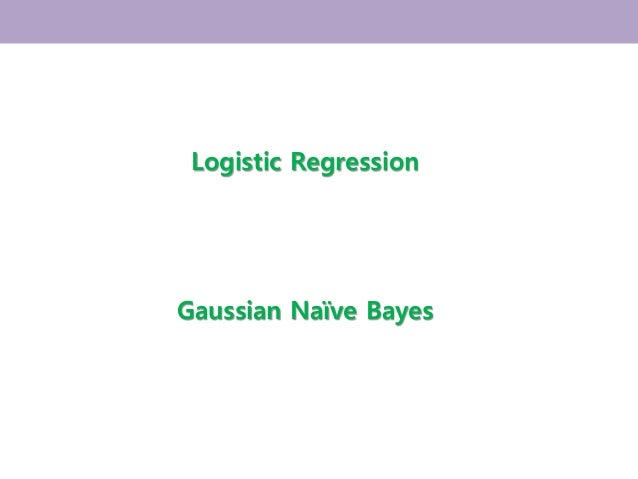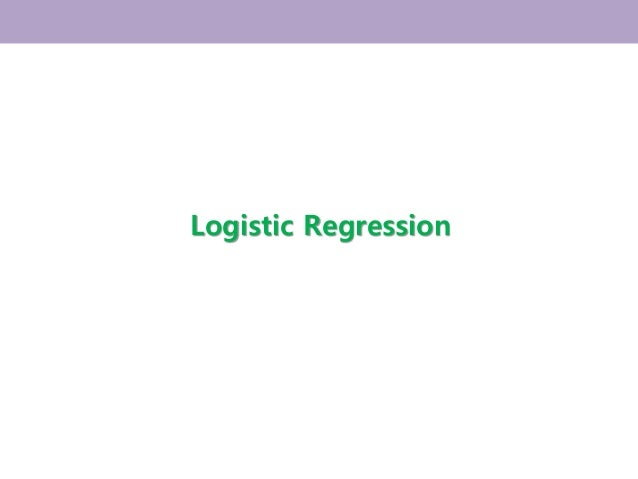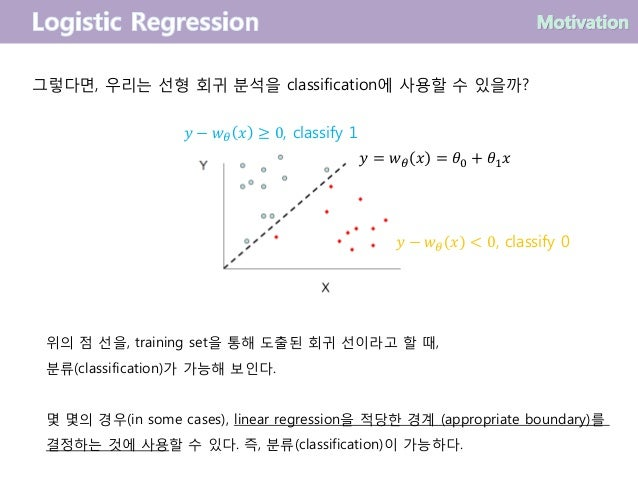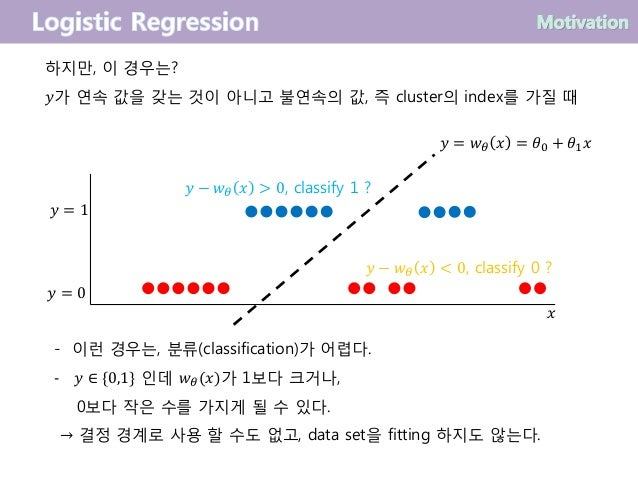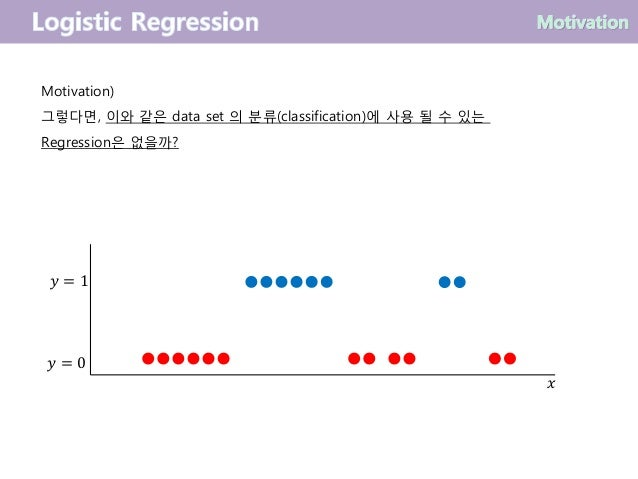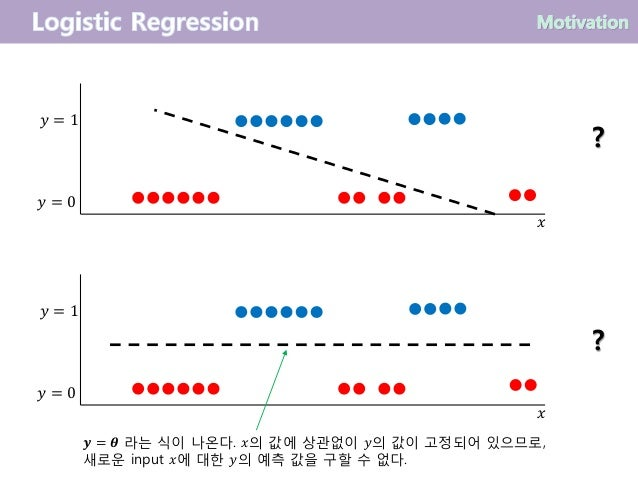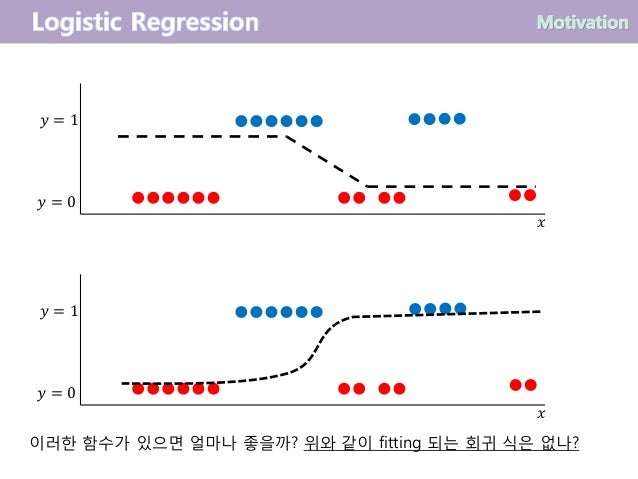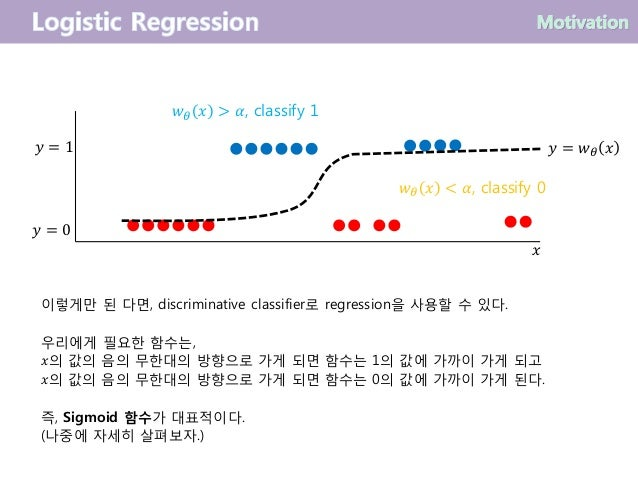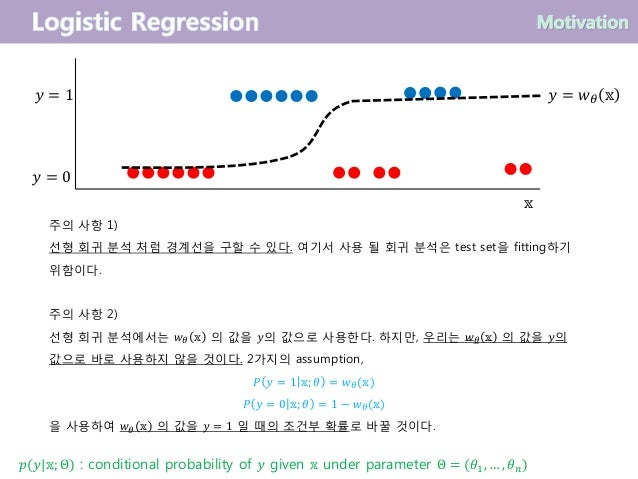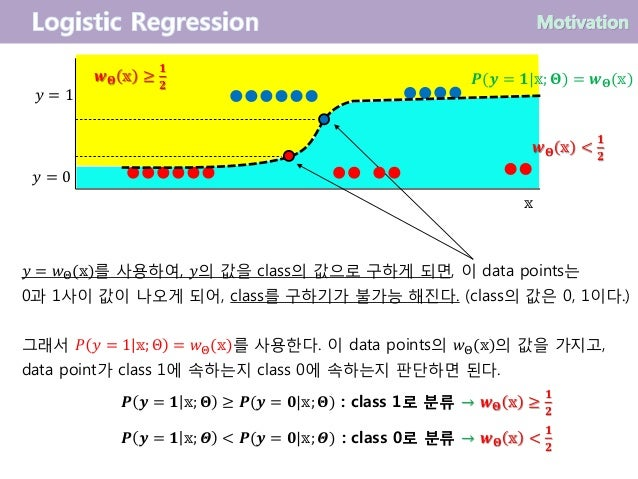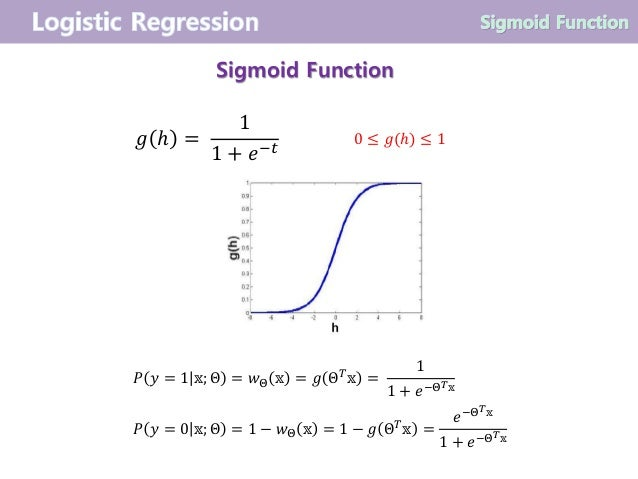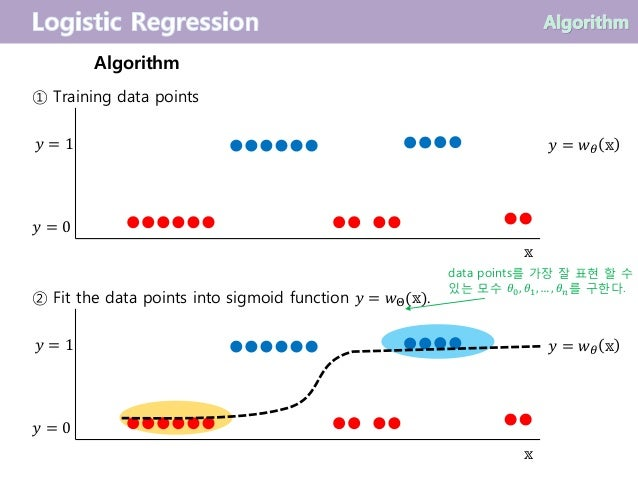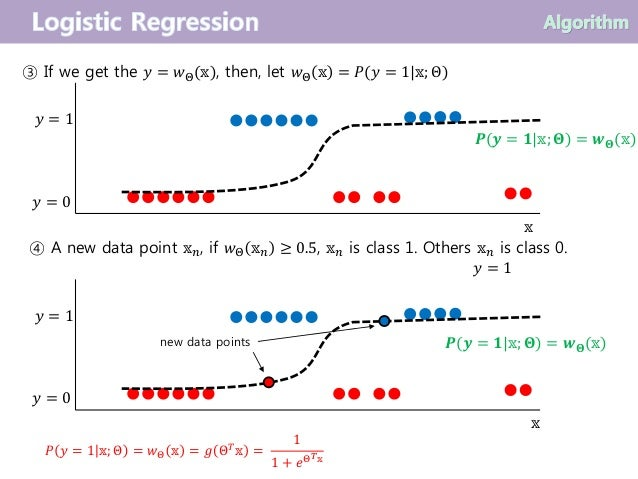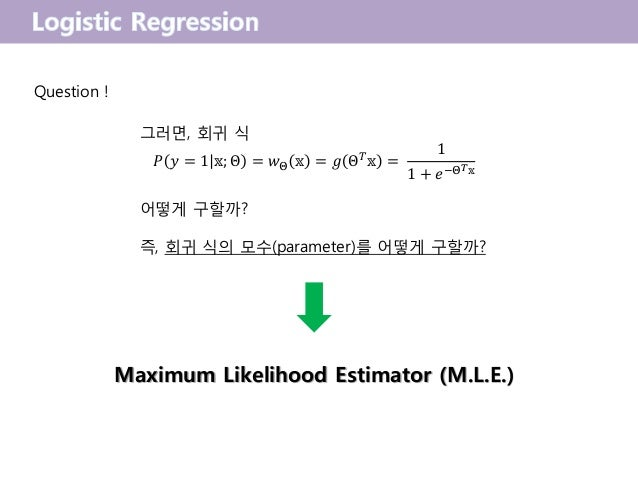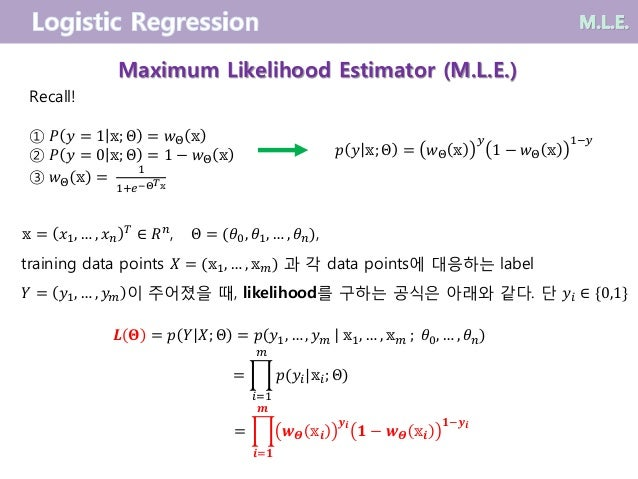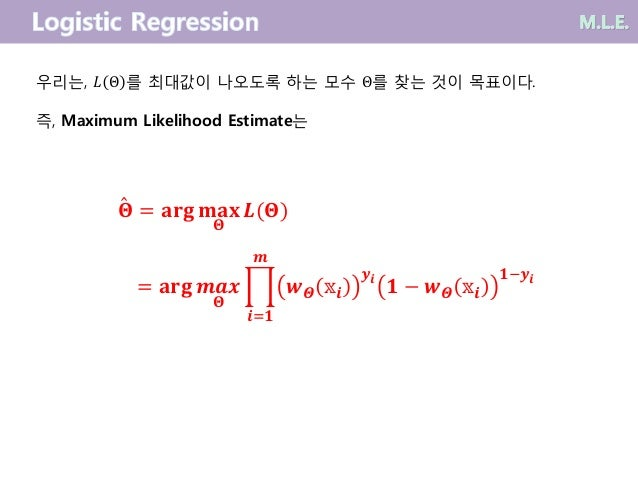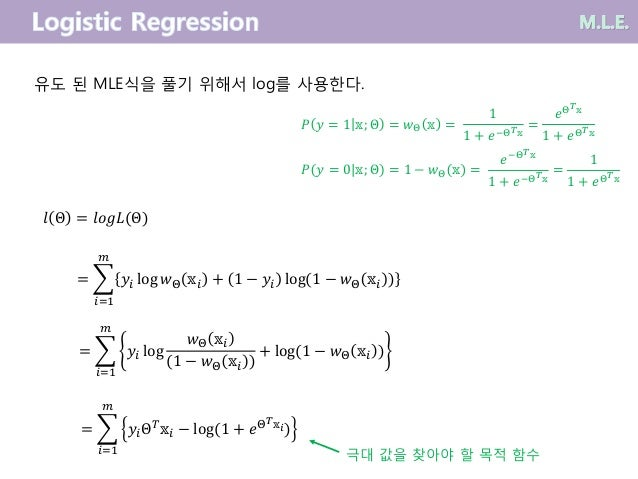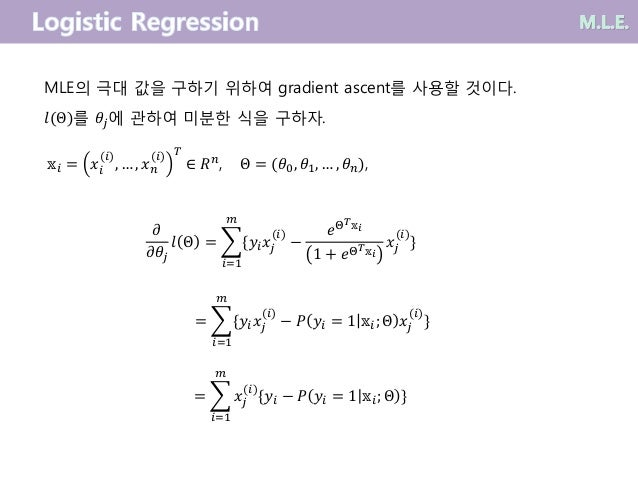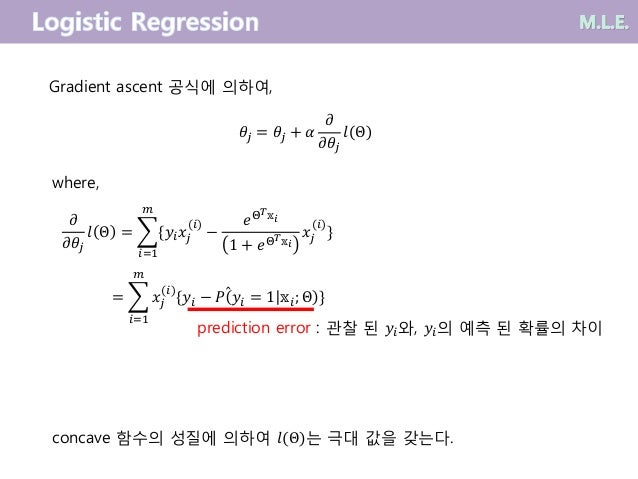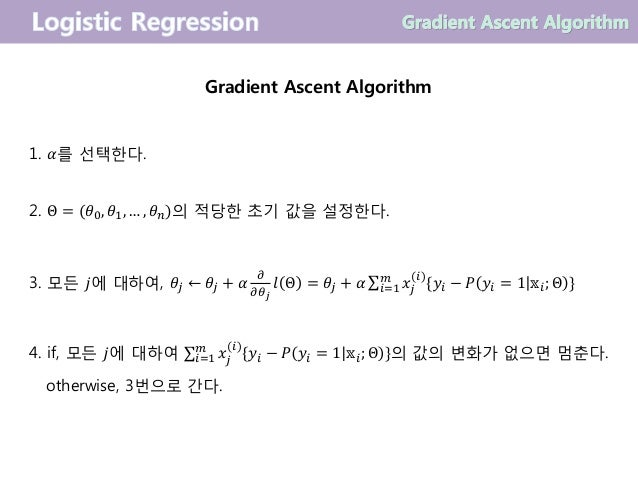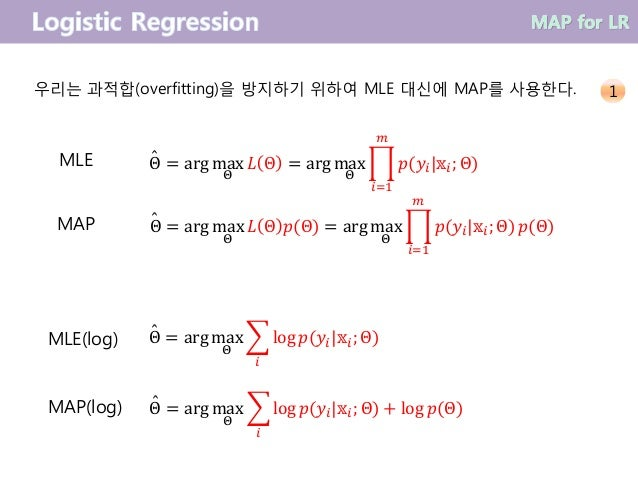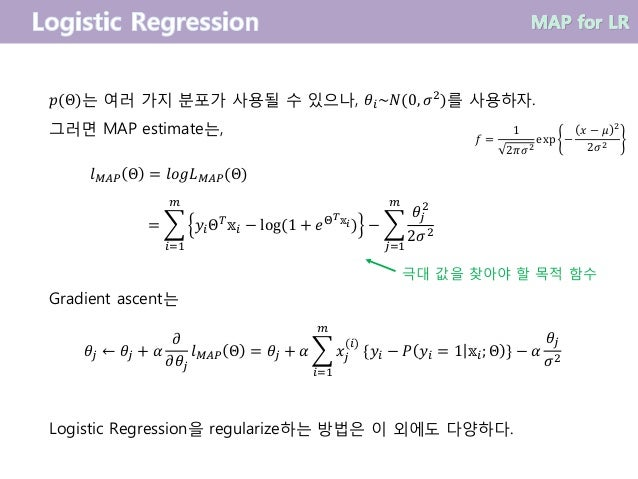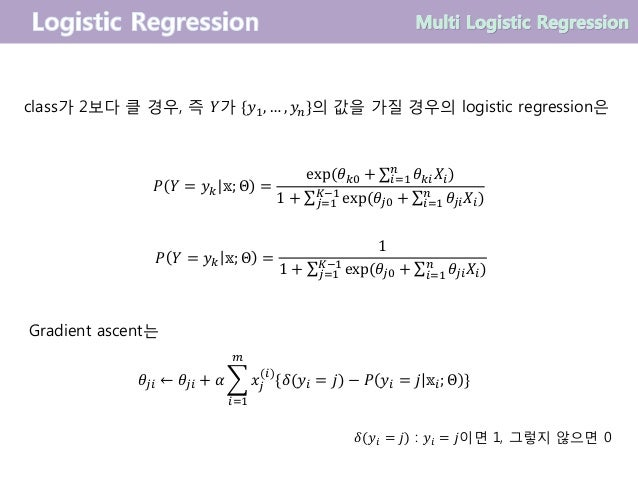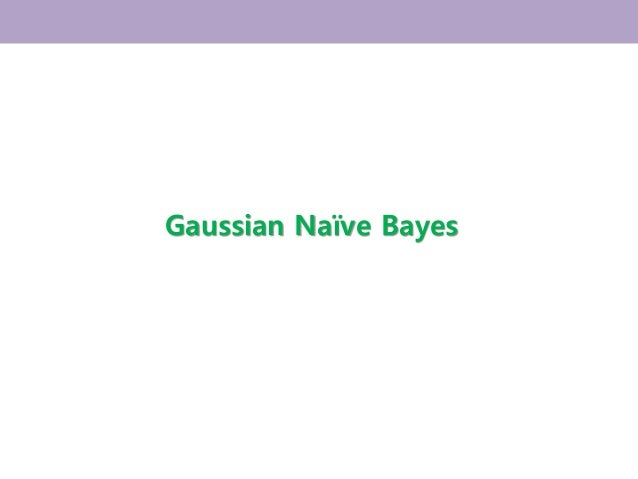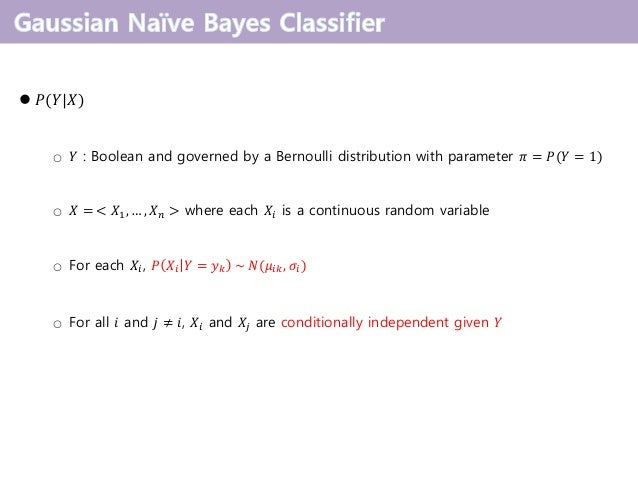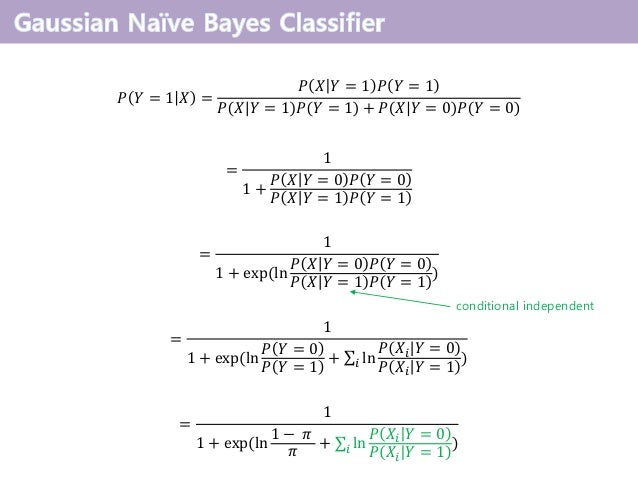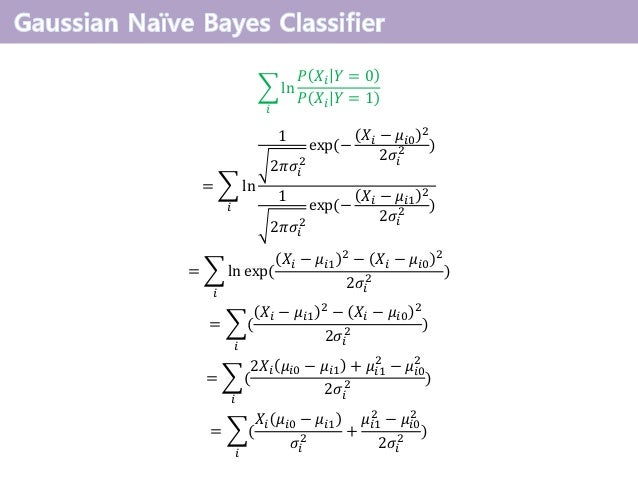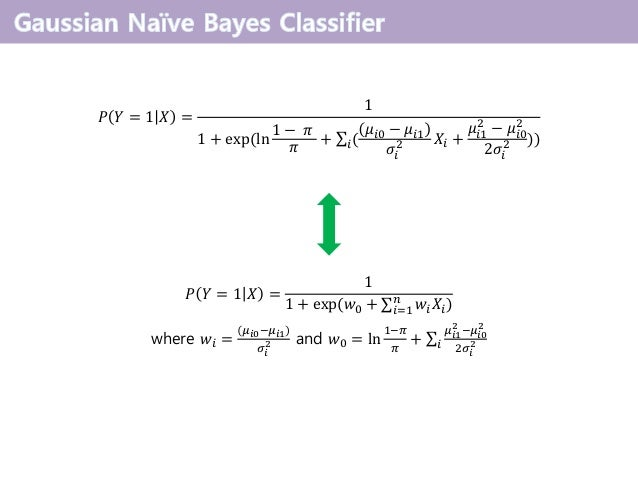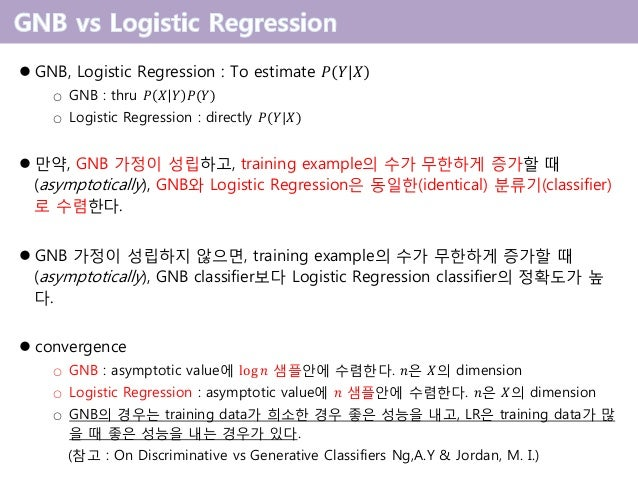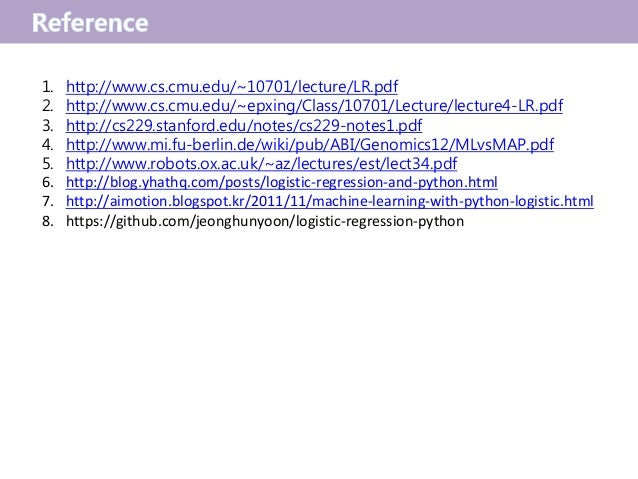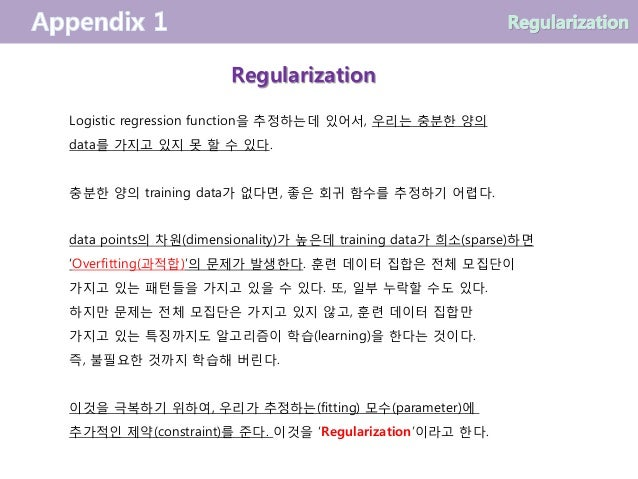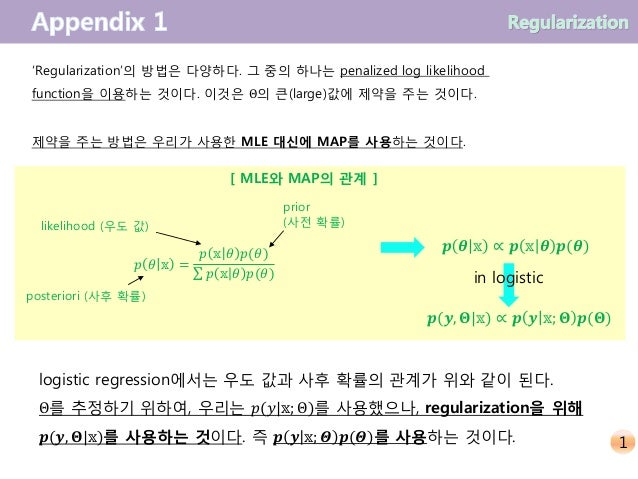Upcoming SlideShare
×

# 04. logistic regression ( 로지스틱 회귀 )

logistic regression in machine learning
( 로지스틱 회귀분석 , 기계학습 )

로지스틱 회귀분석에 관한 내용을 알기 쉽게 설명한 글이다.
또한 MLE, MAP에 대한 설명과 정규화(regularization)에 대한 설명을 해놓은 글이다.

• Full Name
Comment goes here.

Are you sure you want to Yes No• Be the first to comment

### 04. logistic regression ( 로지스틱 회귀 )

1. 1. Jeonghun Yoon
2. 2. Question : 2개의 cluster로 나누고 싶다. How?
3. 3. Training set을 이용하여, 군집(cluster) 전체의 모델을 찾기 원한다. 우리는 군집(cluster)의 확률 모델을 알고 있기 때문에, 새로운 데이터가 들어올 경우, 군집의 확률 모델을 다시 만들 수 있다. Training set으로 부터 𝑃(𝑋|𝑌), 𝑃(𝑌)를 바로 추정한다. 대표적인 예는 Naive Bayes classifier 이다. Generative Classifier 생성하는 𝑃(𝑌|𝑋) 𝑃 𝑋 𝑌 , 𝑃(𝑌) Generative
4. 4. Training set을 이용하여, 군집을 나누어 줄 수 있는 경계선을 찾길 원한다. Clusters를 구별할 수 있는 boundary(경계)에 있는 data가 중요하다. Training set으로 부터 𝑃 𝑌 𝑋 를 바로 추정한다. 가장 대표적인 예는 SVM, Logistic Classifier이다. Discriminative Classifier
5. 5. Logistic Regression Gaussian Naïve Bayes
6. 6. Logistic Regression
7. 7. 그렇다면, 우리는 선형 회귀 분석을 classification에 사용할 수 있을까? 위의 점 선을, training set을 통해 도출된 회귀 선이라고 할 때, 분류(classification)가 가능해 보인다. 몇 몇의 경우(in some cases), linear regression을 적당한 경계 (appropriate boundary)를 결정하는 것에 사용할 수 있다. 즉, 분류(classification)이 가능하다. 𝑦 = 𝑤 𝜃 𝑥 = 𝜃0 + 𝜃1 𝑥 𝑦 − 𝑤 𝜃 𝑥 ≥ 0, classify 1 𝑦 − 𝑤 𝜃 𝑥 < 0, classify 0
8. 8. 하지만, 이 경우는? 𝑦가 연속 값을 갖는 것이 아니고 불연속의 값, 즉 cluster의 index를 가질 때 𝑦 − 𝑤 𝜃 𝑥 > 0, classify 1 ? 𝑦 − 𝑤 𝜃 𝑥 < 0, classify 0 ? - 이런 경우는, 분류(classification)가 어렵다. 𝑦- ∈ {0,1} 인데 𝑤 𝜃(𝑥)가 1보다 크거나, 0보다 작은 수를 가지게 될 수 있다. → 결정 경계로 사용 할 수도 없고, data set을 fitting 하지도 않는다. 𝑦 = 𝑤 𝜃 𝑥 = 𝜃0 + 𝜃1 𝑥 𝑦 = 0 𝑦 = 1 𝑥
9. 9. Motivation) 그렇다면, 이와 같은 data set 의 분류(classification)에 사용 될 수 있는 Regression은 없을까? 𝑦 = 0 𝑦 = 1 𝑥
10. 10. 𝑦 = 0 𝑦 = 1 𝑥 ? 𝑦 = 0 𝑦 = 1 𝑥 ? 𝒚 = 𝜽 라는 식이 나온다. 𝑥의 값에 상관없이 𝑦의 값이 고정되어 있으므로, 새로운 input 𝑥에 대한 𝑦의 예측 값을 구할 수 없다.
11. 11. 𝑦 = 0 𝑦 = 1 𝑥 𝑦 = 0 𝑦 = 1 𝑥 이러한 함수가 있으면 얼마나 좋을까? 위와 같이 fitting 되는 회귀 식은 없나?
12. 12. 이렇게만 된 다면, discriminative classifier로 regression을 사용할 수 있다. 우리에게 필요한 함수는, 𝑥의 값의 음의 무한대의 방향으로 가게 되면 함수는 1의 값에 가까이 가게 되고 𝑥의 값의 음의 무한대의 방향으로 가게 되면 함수는 0의 값에 가까이 가게 된다. 즉, Sigmoid 함수가 대표적이다. (나중에 자세히 살펴보자.) 𝑦 = 0 𝑦 = 1 𝑥 𝑦 = 𝑤 𝜃 𝑥 𝑤 𝜃 𝑥 > 𝛼, classify 1 𝑤 𝜃 𝑥 < 𝛼, classify 0
13. 13. 주의 사항 1) 선형 회귀 분석 처럼 경계선을 구할 수 있다. 여기서 사용 될 회귀 분석은 test set을 fitting하기 위함이다. 주의 사항 2) 선형 회귀 분석에서는 𝑤 𝜃 𝕩 의 값을 𝑦의 값으로 사용한다. 하지만, 우리는 𝑤 𝜃 𝕩 의 값을 𝑦의 값으로 바로 사용하지 않을 것이다. 2가지의 assumption, 𝑃 𝑦 = 1 𝕩; 𝜃 = 𝑤 𝜃(𝕩) 𝑃 𝑦 = 0 𝕩; 𝜃 = 1 − 𝑤 𝜃(𝕩) 을 사용하여 𝑤 𝜃 𝕩 의 값을 𝑦 = 1 일 때의 조건부 확률로 바꿀 것이다. 𝕩 𝑝(𝑦|𝕩; Θ) : conditional probability of 𝑦 given 𝕩 under parameter Θ = (𝜃1, … , 𝜃 𝑛) 𝑦 = 0 𝑦 = 1 𝑦 = 𝑤 𝜃 𝕩
14. 14. 𝑦 = 𝑤Θ(𝕩)를 사용하여, 𝑦의 값을 class의 값으로 구하게 되면, 이 data points는 0과 1사이 값이 나오게 되어, class를 구하기가 불가능 해진다. (class의 값은 0, 1이다.) 그래서 𝑃 𝑦 = 1 𝕩; Θ = 𝑤Θ(𝕩)를 사용한다. 이 data points의 𝑤Θ(𝕩)의 값을 가지고, data point가 class 1에 속하는지 class 0에 속하는지 판단하면 된다. 𝑷 𝒚 = 𝟏 𝕩; 𝚯 ≥ 𝑷(𝒚 = 𝟎|𝕩; 𝚯) : class 1로 분류 → 𝒘 𝚯 𝕩 ≥ 𝟏 𝟐 𝑷 𝒚 = 𝟏 𝕩; 𝜣 < 𝑷(𝒚 = 𝟎|𝕩; 𝜣) : class 0로 분류 → 𝒘 𝚯 𝕩 < 𝟏 𝟐 𝑷(𝒚 = 𝟏|𝕩; 𝚯) = 𝒘 𝚯(𝕩) 𝕩 𝑦 = 0 𝑦 = 1 𝒘 𝚯 𝕩 ≥ 𝟏 𝟐 𝒘 𝚯 𝕩 < 𝟏 𝟐
15. 15. Sigmoid Function 𝑔 ℎ = 1 1 + 𝑒−𝑡 0 ≤ 𝑔(ℎ) ≤ 1 𝑃 𝑦 = 1 𝕩; Θ = 𝑤Θ 𝕩 = 𝑔 Θ 𝑇 𝕩 = 1 1 + 𝑒−Θ 𝑇 𝕩 𝑃 𝑦 = 0 𝕩; Θ = 1 − 𝑤Θ 𝕩 = 1 − 𝑔 Θ 𝑇 𝕩 = 𝑒−Θ 𝑇 𝕩 1 + 𝑒−Θ 𝑇 𝕩
16. 16. ① Training data points ② Fit the data points into sigmoid function 𝑦 = 𝑤Θ(𝕩). Algorithm data points를 가장 잘 표현 할 수 있는 모수 𝜃0, 𝜃1, … , 𝜃 𝑛를 구한다. 𝕩 𝑦 = 0 𝑦 = 1 𝑦 = 𝑤 𝜃 𝕩 𝕩 𝑦 = 0 𝑦 = 1 𝑦 = 𝑤 𝜃 𝕩
17. 17. 𝑷(𝒚 = 𝟏|𝕩; 𝚯) = 𝒘 𝚯(𝕩) ③ If we get the 𝑦 = 𝑤Θ(𝕩), then, let 𝑤Θ 𝕩 = 𝑃(𝑦 = 1|𝕩; Θ) 𝕩 𝑦 = 1 𝑷(𝒚 = 𝟏|𝕩; 𝚯) = 𝒘 𝚯(𝕩) ④ A new data point 𝕩 𝑛, if 𝑤Θ 𝕩 𝑛 ≥ 0.5, 𝕩 𝑛 is class 1. Others 𝕩 𝑛 is class 0. new data points 𝑃 𝑦 = 1 𝕩; Θ = 𝑤Θ 𝕩 = 𝑔 Θ 𝑇 𝕩 = 1 1 + 𝑒Θ 𝑇 𝕩 𝕩 𝑦 = 0 𝑦 = 1 𝑦 = 0 𝑦 = 1
18. 18. 그러면, 회귀 식 𝑃 𝑦 = 1 𝕩; Θ = 𝑤Θ 𝕩 = 𝑔 Θ 𝑇 𝕩 = 1 1 + 𝑒−Θ 𝑇 𝕩 어떻게 구할까? 즉, 회귀 식의 모수(parameter)를 어떻게 구할까? Maximum Likelihood Estimator (M.L.E.) Question !
19. 19. Maximum Likelihood Estimator (M.L.E.) Recall! ① 𝑃 𝑦 = 1 𝕩; Θ = 𝑤Θ 𝕩 ② 𝑃 𝑦 = 0 𝕩; Θ = 1 − 𝑤Θ 𝕩 ③ 𝑤Θ 𝕩 = 1 1+𝑒−Θ 𝑇 𝕩 𝑝 𝑦 𝕩; Θ = 𝑤Θ 𝕩 𝑦 1 − 𝑤Θ 𝕩 1−𝑦 training data points 𝑋 = (𝕩1, … , 𝕩 𝑚) 과 각 data points에 대응하는 label 𝑌 = 𝑦1, … , 𝑦 𝑚 이 주어졌을 때, likelihood를 구하는 공식은 아래와 같다. 단 𝑦𝑖 ∈ {0,1} 𝑳 𝚯 = 𝑝 𝑌 𝑋; Θ = 𝑝 𝑦1, … , 𝑦 𝑚 𝕩1, … , 𝕩 𝑚 ; 𝜃0, … , 𝜃 𝑛) 𝕩 = 𝑥1, … , 𝑥 𝑛 𝑇 ∈ 𝑅 𝑛 , Θ = (𝜃0, 𝜃1, … , 𝜃 𝑛), = ෑ 𝑖=1 𝑚 𝑝(𝑦𝑖|𝕩𝑖; Θ) = ෑ 𝒊=𝟏 𝒎 𝒘 𝜣 𝕩𝒊 𝒚 𝒊 𝟏 − 𝒘 𝜣 𝕩𝒊 𝟏−𝒚𝒊
20. 20. 우리는, 𝐿 Θ 를 최대값이 나오도록 하는 모수 Θ를 찾는 것이 목표이다. 즉, Maximum Likelihood Estimate는 𝚯 = 𝐚𝐫𝐠 𝐦𝐚𝐱 𝚯 𝑳(𝚯) = 𝐚𝐫𝐠 𝒎𝒂𝒙 𝚯 ෑ 𝒊=𝟏 𝒎 𝒘 𝜣 𝕩𝒊 𝒚 𝒊 𝟏 − 𝒘 𝜣 𝕩𝒊 𝟏−𝒚 𝒊 ̂
21. 21. 𝑙 Θ = 𝑙𝑜𝑔𝐿(Θ) 유도 된 MLE식을 풀기 위해서 log를 사용한다. 𝑃 𝑦 = 1 𝕩; Θ = 𝑤Θ 𝕩 = 1 1 + 𝑒−Θ 𝑇 𝕩 = 𝑒Θ 𝑇 𝕩 1 + 𝑒Θ 𝑇 𝕩 𝑃 𝑦 = 0 𝕩; Θ = 1 − 𝑤Θ 𝕩 = 𝑒−Θ 𝑇 𝕩 1 + 𝑒−Θ 𝑇 𝕩 = 1 1 + 𝑒Θ 𝑇 𝕩 = ෍ 𝑖=1 𝑚 𝑦𝑖 log 𝑤Θ 𝕩𝑖 + 1 − 𝑦𝑖 log(1 − 𝑤Θ 𝕩𝑖 ) = ෍ 𝑖=1 𝑚 𝑦𝑖 log 𝑤Θ 𝕩𝑖 (1 − 𝑤Θ 𝕩𝑖 ) + log(1 − 𝑤Θ 𝕩𝑖 ) = ෍ 𝑖=1 𝑚 𝑦𝑖Θ 𝑇 𝕩𝑖 − log(1 + 𝑒Θ 𝑇 𝕩𝑖) 극대 값을 찾아야 할 목적 함수
22. 22. MLE의 극대 값을 구하기 위하여 gradient ascent를 사용할 것이다. 𝑙(Θ)를 𝜃𝑗에 관하여 미분한 식을 구하자. 𝜕 𝜕𝜃𝑗 𝑙 Θ = ෍ 𝑖=1 𝑚 {𝑦𝑖 𝑥𝑗 (𝑖) − 𝑒Θ 𝑇 𝕩𝑖 1 + 𝑒Θ 𝑇 𝕩𝑖 𝑥𝑗 (𝑖) } 𝕩𝑖 = 𝑥𝑖 (𝑖) , … , 𝑥 𝑛 (𝑖) 𝑇 ∈ 𝑅 𝑛, Θ = (𝜃0, 𝜃1, … , 𝜃 𝑛), = ෍ 𝑖=1 𝑚 {𝑦𝑖 𝑥𝑗 (𝑖) − 𝑃 𝑦𝑖 = 1 𝕩𝑖; Θ 𝑥𝑗 (𝑖) } = ෍ 𝑖=1 𝑚 𝑥𝑗 (𝑖) {𝑦𝑖 − 𝑃 𝑦𝑖 = 1 𝕩𝑖; Θ }
23. 23. 𝜕 𝜕𝜃𝑗 𝑙 Θ = ෍ 𝑖=1 𝑚 {𝑦𝑖 𝑥𝑗 (𝑖) − 𝑒Θ 𝑇 𝕩𝑖 1 + 𝑒Θ 𝑇 𝕩𝑖 𝑥𝑗 (𝑖) } = ෍ 𝑖=1 𝑚 𝑥𝑗 (𝑖) {𝑦𝑖 − 𝑃 𝑦𝑖 = 1 𝕩𝑖; Θ } prediction error : 관찰 된 𝑦𝑖와, 𝑦𝑖의 예측 된 확률의 차이 ˆ 𝜃𝑗 = 𝜃𝑗 + 𝛼 𝜕 𝜕𝜃𝑗 𝑙 Θ Gradient ascent 공식에 의하여, where, concave 함수의 성질에 의하여 𝑙(Θ)는 극대 값을 갖는다.
24. 24. Gradient Ascent Algorithm 1. 𝛼를 선택한다. 2. Θ = (𝜃0, 𝜃1, … , 𝜃 𝑛)의 적당한 초기 값을 설정한다. 3. 모든 𝑗에 대하여, 𝜃𝑗 ← 𝜃𝑗 + 𝛼 𝜕 𝜕𝜃 𝑗 𝑙 Θ = 𝜃𝑗 + 𝛼 σ𝑖=1 𝑚 𝑥𝑗 (𝑖) {𝑦𝑖 − 𝑃 𝑦𝑖 = 1 𝕩𝑖; Θ } 4. if, 모든 𝑗에 대하여 σ𝑖=1 𝑚 𝑥𝑗 (𝑖) {𝑦𝑖 − 𝑃 𝑦𝑖 = 1 𝕩𝑖; Θ }의 값의 변화가 없으면 멈춘다. otherwise, 3번으로 간다.
25. 25. Θ = arg max Θ 𝐿 Θ = arg max Θ ෑ 𝑖=1 𝑚 𝑝(𝑦𝑖|𝕩𝑖; Θ) Θ = arg max Θ 𝐿 Θ 𝑝(Θ) = arg max Θ ෑ 𝑖=1 𝑚 𝑝(𝑦𝑖|𝕩𝑖; Θ) 𝑝(Θ) Θ = arg max Θ ෍ 𝑖 log 𝑝(𝑦𝑖|𝕩𝑖; Θ) Θ = arg max Θ ෍ 𝑖 log 𝑝(𝑦𝑖|𝕩𝑖; Θ) + log 𝑝(Θ) MLE MAP MLE(log) MAP(log) ̂ ̂ ̂ ̂ 우리는 과적합(overfitting)을 방지하기 위하여 MLE 대신에 MAP를 사용한다. 1
26. 26. 𝑝(Θ)는 여러 가지 분포가 사용될 수 있으나, 𝜃𝑖~𝑁(0, 𝜎2)를 사용하자. 그러면 MAP estimate는, 𝑙 𝑀𝐴𝑃 Θ = 𝑙𝑜𝑔𝐿 𝑀𝐴𝑃(Θ) = ෍ 𝑖=1 𝑚 𝑦𝑖Θ 𝑇 𝕩𝑖 − log(1 + 𝑒Θ 𝑇 𝕩𝑖) − ෍ 𝑗=1 𝑚 𝜃𝑗 2 2𝜎2 Gradient ascent는 𝜃𝑗 ← 𝜃𝑗 + 𝛼 𝜕 𝜕𝜃𝑗 𝑙 𝑀𝐴𝑃 Θ = 𝜃𝑗 + 𝛼 ෍ 𝑖=1 𝑚 𝑥𝑗 (𝑖) {𝑦𝑖 − 𝑃 𝑦𝑖 = 1 𝕩𝑖; Θ } − 𝛼 𝜃𝑗 𝜎2 𝑓 = 1 2𝜋𝜎2 exp − 𝑥 − 𝜇 2 2𝜎2 Logistic Regression을 regularize하는 방법은 이 외에도 다양하다. 극대 값을 찾아야 할 목적 함수
27. 27. class가 2보다 클 경우, 즉 𝑌가 {𝑦1, … , 𝑦 𝑛}의 값을 가질 경우의 logistic regression은 𝑃 𝑌 = 𝑦 𝑘 𝕩; Θ = exp(𝜃 𝑘0 + σ𝑖=1 𝑛 𝜃 𝑘𝑖 𝑋𝑖) 1 + σ 𝑗=1 𝐾−1 exp(𝜃𝑗0 + σ𝑖=1 𝑛 𝜃𝑗𝑖 𝑋𝑖) 𝑃 𝑌 = 𝑦 𝑘 𝕩; Θ = 1 1 + σ 𝑗=1 𝐾−1 exp(𝜃𝑗0 + σ𝑖=1 𝑛 𝜃𝑗𝑖 𝑋𝑖) Gradient ascent는 𝜃𝑗𝑖 ← 𝜃𝑗𝑖 + 𝛼 ෍ 𝑖=1 𝑚 𝑥𝑗 (𝑖) {𝛿(𝑦𝑖 = 𝑗) − 𝑃 𝑦𝑖 = 𝑗 𝕩𝑖; Θ } 𝛿(𝑦𝑖 = 𝑗) : 𝑦𝑖 = 𝑗이면 1, 그렇지 않으면 0
28. 28. Gaussian Naïve Bayes
29. 29. 𝑃 (𝑌|𝑋) 𝑌○ : Boolean and governed by a Bernoulli distribution with parameter 𝜋 = 𝑃(𝑌 = 1) 𝑋○ = < 𝑋1, … , 𝑋 𝑛 > where each 𝑋𝑖 is a continuous random variable For each○ 𝑋𝑖, 𝑃 𝑋𝑖 𝑌 = 𝑦 𝑘 ~ 𝑁(𝜇𝑖𝑘, 𝜎𝑖) For all○ 𝑖 and 𝑗 ≠ 𝑖, 𝑋𝑖 and 𝑋𝑗 are conditionally independent given 𝑌
30. 30. 𝑃 𝑌 = 1 𝑋 = 𝑃 𝑋 𝑌 = 1 𝑃 𝑌 = 1 𝑃 𝑋 𝑌 = 1 𝑃 𝑌 = 1 + 𝑃 𝑋 𝑌 = 0 𝑃 𝑌 = 0 = 1 1 + 𝑃 𝑋 𝑌 = 0 𝑃 𝑌 = 0 𝑃 𝑋 𝑌 = 1 𝑃 𝑌 = 1 = 1 1 + exp(ln 𝑃 𝑋 𝑌 = 0 𝑃 𝑌 = 0 𝑃 𝑋 𝑌 = 1 𝑃 𝑌 = 1 ) = 1 1 + exp(ln 𝑃 𝑌 = 0 𝑃 𝑌 = 1 + σ𝑖 ln 𝑃 𝑋𝑖 𝑌 = 0 𝑃 𝑋𝑖 𝑌 = 1 ) = 1 1 + exp(ln 1 − 𝜋 𝜋 + σ𝑖 ln 𝑃 𝑋𝑖 𝑌 = 0 𝑃 𝑋𝑖 𝑌 = 1 ) conditional independent
31. 31. ෍ 𝑖 ln 𝑃 𝑋𝑖 𝑌 = 0 𝑃 𝑋𝑖 𝑌 = 1 = ෍ 𝑖 ln 1 2𝜋𝜎𝑖 2 exp(− 𝑋𝑖 − 𝜇𝑖0 2 2𝜎𝑖 2 ) 1 2𝜋𝜎𝑖 2 exp(− 𝑋𝑖 − 𝜇𝑖1 2 2𝜎𝑖 2 ) = ෍ 𝑖 ln exp( 𝑋𝑖 − 𝜇𝑖1 2 − 𝑋𝑖 − 𝜇𝑖0 2 2𝜎𝑖 2 ) = ෍ 𝑖 ( 𝑋𝑖 − 𝜇𝑖1 2 − 𝑋𝑖 − 𝜇𝑖0 2 2𝜎𝑖 2 ) = ෍ 𝑖 ( 2𝑋𝑖 𝜇𝑖0 − 𝜇𝑖1 + 𝜇𝑖1 2 − 𝜇𝑖0 2 2𝜎𝑖 2 ) = ෍ 𝑖 ( 𝑋𝑖 𝜇𝑖0 − 𝜇𝑖1 𝜎𝑖 2 + 𝜇𝑖1 2 − 𝜇𝑖0 2 2𝜎𝑖 2 )
32. 32. 𝑃 𝑌 = 1 𝑋 = 1 1 + exp(ln 1 − 𝜋 𝜋 + σ𝑖( 𝜇𝑖0 − 𝜇𝑖1 𝜎𝑖 2 𝑋𝑖 + 𝜇𝑖1 2 − 𝜇𝑖0 2 2𝜎𝑖 2 )) 𝑃 𝑌 = 1 𝑋 = 1 1 + exp(𝑤0 + σ𝑖=1 𝑛 𝑤𝑖 𝑋𝑖) where 𝑤𝑖 = 𝜇 𝑖0−𝜇 𝑖1 𝜎𝑖 2 and 𝑤0 = ln 1−𝜋 𝜋 + σ𝑖 𝜇 𝑖1 2 −𝜇 𝑖0 2 2𝜎𝑖 2
33. 33. GNB, Logistic Regression : To estimate 𝑃 𝑌 𝑋 GNB : thru○ 𝑃 𝑋 𝑌 𝑃(𝑌) Logistic Regression : directly○ 𝑃(𝑌|𝑋) 만약 , GNB 가정이 성립하고, training example의 수가 무한하게 증가할 때 (asymptotically), GNB와 Logistic Regression은 동일한(identical) 분류기(classifier) 로 수렴한다. GNB 가정이 성립하지 않으면, training example의 수가 무한하게 증가할 때 (asymptotically), GNB classifier보다 Logistic Regression classifier의 정확도가 높 다. convergence GNB○ : asymptotic value에 log 𝑛 샘플안에 수렴한다. 𝑛은 𝑋의 dimension Logistic Regression○ : asymptotic value에 𝑛 샘플안에 수렴한다. 𝑛은 𝑋의 dimension GNB○ 의 경우는 training data가 희소한 경우 좋은 성능을 내고, LR은 training data가 많 을 때 좋은 성능을 내는 경우가 있다. (참고 : On Discriminative vs Generative Classifiers Ng,A.Y & Jordan, M. I.)
34. 34. http://1. www.cs.cmu.edu/~10701/lecture/LR.pdf http://2. www.cs.cmu.edu/~epxing/Class/10701/Lecture/lecture4-LR.pdf http://3. cs229.stanford.edu/notes/cs229-notes1.pdf http://4. www.mi.fu-berlin.de/wiki/pub/ABI/Genomics12/MLvsMAP.pdf http://5. www.robots.ox.ac.uk/~az/lectures/est/lect34.pdf http://6. blog.yhathq.com/posts/logistic-regression-and-python.html http7. ://aimotion.blogspot.kr/2011/11/machine-learning-with-python-logistic.html https://github.com/jeonghunyoon/logistic8. -regression-python
35. 35. Regularization Logistic regression function을 추정하는데 있어서, 우리는 충분한 양의 data를 가지고 있지 못 할 수 있다. 충분한 양의 training data가 없다면, 좋은 회귀 함수를 추정하기 어렵다. data points의 차원(dimensionality)가 높은데 training data가 희소(sparse)하면 ‘Overfitting(과적합)’의 문제가 발생한다. 훈련 데이터 집합은 전체 모집단이 가지고 있는 패턴들을 가지고 있을 수 있다. 또, 일부 누락할 수도 있다. 하지만 문제는 전체 모집단은 가지고 있지 않고, 훈련 데이터 집합만 가지고 있는 특징까지도 알고리즘이 학습(learning)을 한다는 것이다. 즉, 불필요한 것까지 학습해 버린다. 이것을 극복하기 위하여, 우리가 추정하는(fitting) 모수(parameter)에 추가적인 제약(constraint)를 준다. 이것을 ‘Regularization’이라고 한다.
36. 36. ‘Regularization’의 방법은 다양하다. 그 중의 하나는 penalized log likelihood function을 이용하는 것이다. 이것은 Θ의 큰(large)값에 제약을 주는 것이다. 제약을 주는 방법은 우리가 사용한 MLE 대신에 MAP를 사용하는 것이다. [ MLE와 MAP의 관계 ] 𝑝 𝜃 𝕩 = 𝑝 𝕩 𝜃 𝑝(𝜃) σ 𝑝 𝕩 𝜃 𝑝(𝜃) posteriori (사후 확률) likelihood (우도 값) prior (사전 확률) 𝒑 𝜽 𝕩 ∝ 𝒑 𝕩 𝜽 𝒑(𝜽) 𝒑(𝒚, 𝚯|𝕩) ∝ 𝒑 𝒚 𝕩; 𝚯 𝒑(𝚯) logistic regression에서는 우도 값과 사후 확률의 관계가 위와 같이 된다. Θ를 추정하기 위하여, 우리는 𝑝(𝑦|𝕩; Θ)를 사용했으나, regularization을 위해 𝒑(𝒚, 𝚯|𝕩)를 사용하는 것이다. 즉 𝒑 𝒚 𝕩; 𝜣 𝒑(𝜣)를 사용하는 것이다. in logistic 1

### Be the first to comment

•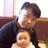#### do3ng1

May. 19, 2018
•#### YuriChoi19

Sep. 6, 2018
•#### ssuserab382e

Dec. 21, 2018

Total views

4,695

On Slideshare

0

From embeds

0

Number of embeds

41

89

Shares

0